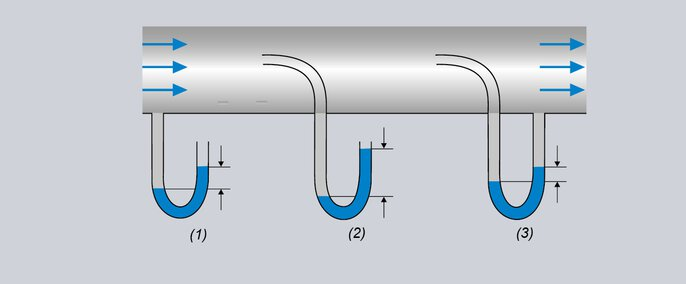Definition

# Static and dynamic pressure in fluids

Fluid is the overarching term for liquids and gases. The physical laws of fluid mechanics apply to both aggregate states. When fluids are in a resting position, i.e. when there is no flow, only the static pressure (p stat) acts on the fluids, evenly in all directions.

As soon as the medium is flowing, for example in a pipe, the conditions become a bit more complicated. The kinetic energy increases the force effect in the direction of flow. If this force meets an object that is located in the flow, a pressure acts on its surface: this is called dynamic pressure (pdyn). This is calculated using the density ρ and the speed v of the medium:

pdyn = ρ/2 ⋅ v2

However, no additional force is detected perpendicular to the flow. Only the static pressure has an effect here. The sum of both pressures is called the total pressure (ptot).

ptot=pstat+pdyn

## For which applications are these pressures relevant?

Static pressure is relevant for most pressure measurements. The exception is measurements of the flow velocity, which are calculated using the dynamic pressure. Application examples include speed measurements for airplanes or the acquisition of wind speed on wind power plants. In both cases, the Prandtl`s pitot tube is used in which the static pressure counteracts the total pressure and as a result, only the dynamic pressure is displayed.Fig. 1: Flow measurement

(1) Pressure probe for measuring the static pressure

(2) Pitot tube for measuring the total pressure

(3) Prandtl’s pitot tube for measuring the dynamic pressure

p + ρgh + (ρ × v2)/2 = constant

The rod p + ρgh is responsible for the static pressure and the rod (ρ × v2)/2 for the dynamic pressure. Since the sum of the two pressures has a constant, unchanging value, one can assume that the pstat pressure decreases when the velocity of the fluid increases. Using the above law, one can calculate, for example, the velocity of the liquid leaving the opening at the bottom of a tank based on the height of the column of liquid in the tank.

### Examples of application

The static pressure in a central heating system is an extremely important component for the correct operation of the system. For example, the static pressure should be lower than the opening pressure of the safety valve and at the same time higher than the water column pressure. Knowing the water column pressure is indispensable to avoid system faults and a reduction in heating output.